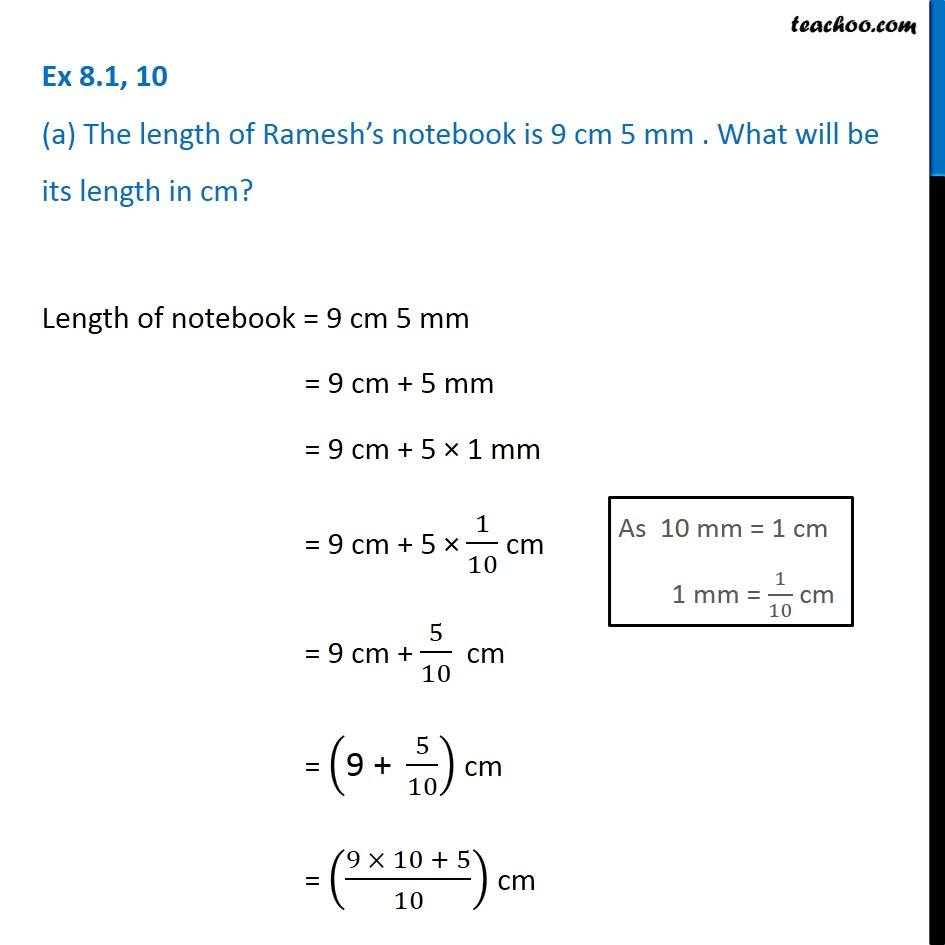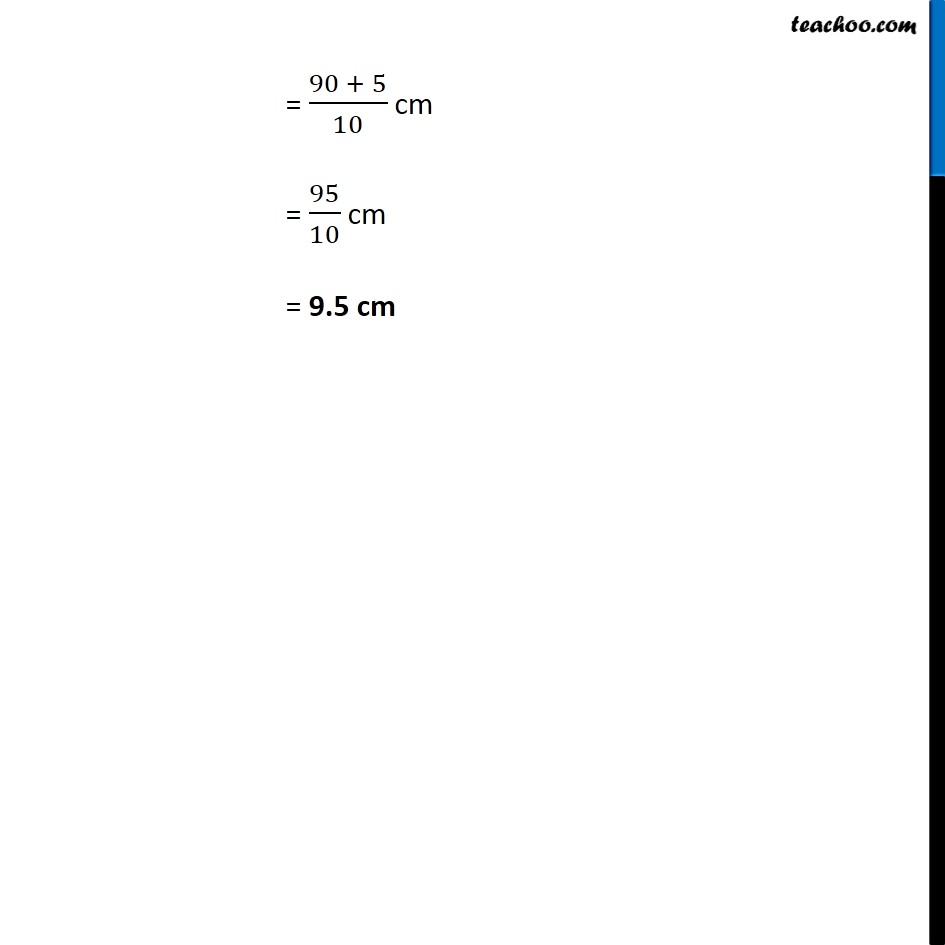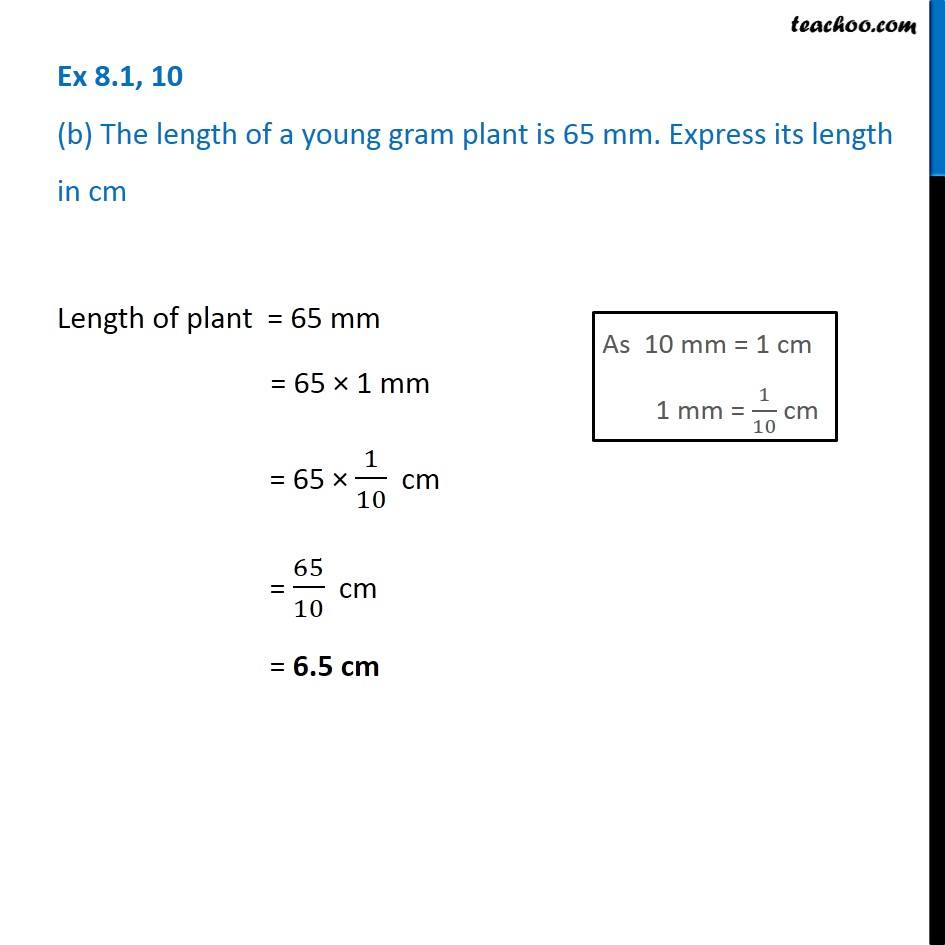Subscribe to our Youtube Channel - https://you.tube/teachoo

1. Chapter 8 Class 6 Decimals
2. Serial order wise
3. Ex 8.1

Transcript

Ex 8.1, 10 (a) The length of Ramesh’s notebook is 9 cm 5 mm . What will be its length in cm? Length of notebook = 9 cm 5 mm = 9 cm + 5 mm = 9 cm + 5 × 1 mm = 9 cm + 5 × 1/10 cm = 9 cm + 5/10 cm = ("9 + " 5/10) cm = ((9 × 10 + 5)/10) cm = (90 + 5)/10 cm = 95/10 cm = 9.5 cm Ex 8.1, 10 (b) The length of a young gram plant is 65 mm. Express its length in cm Length of plant = 65 mm = 65 × 1 mm = 65 × 1/10 cm = 65/10 cm = 6.5 cm

Ex 8.1

Chapter 8 Class 6 Decimals
Serial order wise